# Magnetic Hole. Pictures.

Magnetic trap is an object, unifying hypothetical objects, connected primarily by magnetic forces: neutron drop/liquid; neutron hole; magnetic hole ...

Magnetic hole is a plane/flat analog of the black hole. The black hole is bounded by gravitational forces, and magnetic hole is bounded by magnetic forces, which are dozens of powers stronger than gravity forces.

It is probable that the microscopic magnetic holes can be created at the collider. Magnetic holes will grow capturing the normal substance. As a result, magnetic holes can destroy the Earth. The Solar System can be transformed in the ring structure, analogues to the ring structure of exploded star SN 1987A.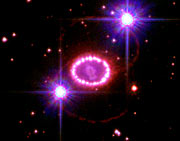The black hole are usually drown in the form of a funnel.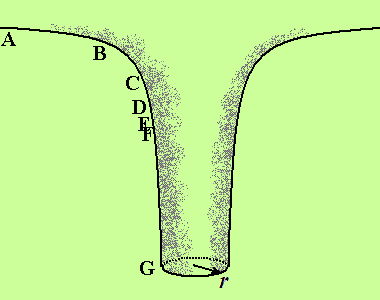That is an approximation. The funnel does not directed everywhere: no to the right, no to the left, no forward, no back... From the point of view of the distant observer, standing a the point A, the time-rate, visible on the watches, placed at points B, C, D, F, E, ..., goes the more slowly, the nearer this point is situated to the event horizon, denoted by the letter G. The distances between every neighbor points of these set are equal, but the visible density of points are growing at our schematic figure. If the observer would drop a stone into the black hole, the stone will never achieve the event horizon. Because the distance from A to G, from the point of view of the observer A is infinite. Or we can say, that the time-rate stops at the event horizon.

If at the center of your room there is a black hole, then the distance from any point of the room to the event horizon of the black hole will be infinite. That can be shown as in the bottom figure.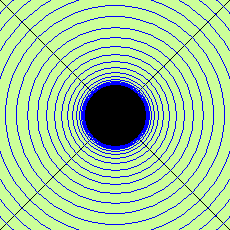Radial distances between every neighbor blue circumferences at the figure are equal, but on the figure we can see these distances unequal. And just near the black hole's horizon the circumferences form one continuous blue ring.

The black hole itself is depicted on the figure by the black circle. There is no space inside the black hole, relatively the external observer, - the black hole is behind the event horizon.

If an apple of mass m would begin to drop into a black hole, it will become smaller and smaller. Gravity influence of an apple itself on observer will be diminishing, but the gravity influence of a funnel will grow correspondingly. We can say, that mass of an apple transforms into another form of matter, into a space-time of a growing gravity hole.

Microscopic black hole, which can be made on Large Hadron Collider can have extremely small radius, smaller than 10-51 m. That is 1035 times smaller than Compton radius of proton. But the mass of such microscopic black hole (mBH) can be greater by several thousands times. It is clear, that such mBH can not swallow the whole proton at once, if we will not assume the hypothesis of quantum capture. From the other hand, we know that proton consists from tree quarks. We can assume, that mBH can try to swallow the quark. It is known that the quark can not exist alone. Consequently, at the time of capture, the thread, connecting the quark and the rest of proton, will broke, and at that place, the pair of quark-antiquark will appear. As a result mBH will swallow the quark-antiquark meson; and the proton can be transformed into a strange matter, if the pair was strange. This idea is also quite hypothetic.

Magnetic holes are much more real and much more dangerous. But at first, let's recollect, what is the physical vacuum? It is known, that a variety of virtual particles and antiparticles are constantly appearing and disappearing in the physical vacuum. These particles are named virtual because of a very short time of their lives. The span of their life-time is limited by the quantum uncertainty principle.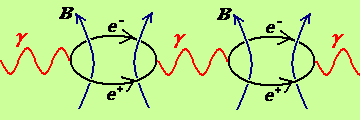You can see at this figure the "virtual chain" of transformations. The events go from the left side to the right. Virtual photon, γ, transforms into a virtual electron-positron pair, e-e+, which after painting a circumference, transforms into virtual photon again. Virtual pair e-e+ creates a magnetic field B, with the configuration, analogues to the field of bar-magnet. Such elemental magnetic dipoles are created spontaneously everywhere and always. Because of their chaotic orientation, the average total magnetic field in the vacuum is equal to zero.

In this article we assumed, that the magnetic hole can be imagined as a set of microscopic circular currents, created by the real charged particles. The magnetic dipole can be observed from its North Pole (blue), or from its South Pole (red), or under some angle.In a space with zero magnetic field, the density of red circles are equal to the density of blue circles. If the density of some circles is greater than other, then in such place we have non-zero magnetic field. If in some place we see only one type of circles, then in this place we have the maximum possible magnetic field.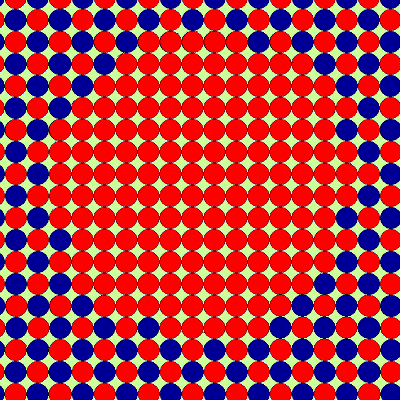At this figure you can see the microscopic magnetic hole.

On the next figure we ca see, how the magnetic hole captures nucleon's restlets. We assumed, that at the time of the capture, the proton ejects the positron and becomes the nucleon's restlet, boson. The same boson is appear at the time of neutron capture, which accompanied by the ejection the antineutrino. The restlets are quanta of force magnetic field, or frozen force lines of the magnetic hole.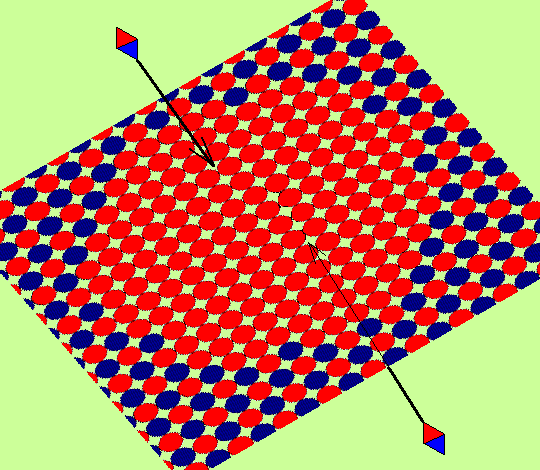Why "frozen"? The dropping of bosons also lasts infinitely long, from the point of view of distant observer, as it was in the case of the black hole. Boson becomes nearer and nearer to the plane of the hole, and makes it wider, constantly increasing its radius.

At the next figure, this idea of the eternally dropping bosons is repeated in the form of the change of the spatial scales, relatively distant observer, looking at the microscopic magnetic dipole, falling at the hole. The scales do not change along the axis x and y. But the z-scales reminds us the behavior of the black hole at the fig. 1. The limiting point G can be situated anywhere in the plane of a blue circle. This circle plays the same role as the sphere of Schwarzschild in the black hole.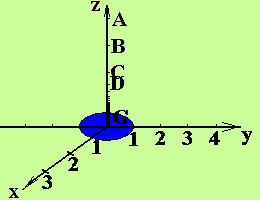If the mass of growing magnetic hole becomes quite big, then the gravity funnel of magnetic hole becomes more essential, and the x- and y-scales are also change.

### Hypothesis.

Gravitational field of the magnetic hole is anisotropic. Let magnetic field is oriented along the z-axis, then the gravity field will be directed along the plane xOy. This can explain, why the majority of galaxies are flattered, and why the peripheral stars of galaxies have abnormally high velocities. On the next figure we can see the approximate directional diagram of the gravitational (black) and magnetic (blue/red) fields of the magnetic hole.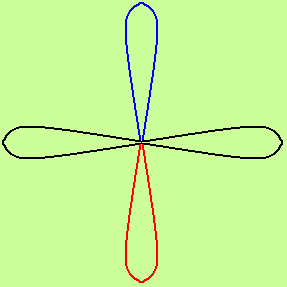Anisotropic gravitational field diminishes more slowly with distance as it was in the case of spherical symmetry. It is very probable, that in the centers of many galaxies there are huge magnetic holes. Such huge hole forms the flattered structure of the galaxy, and can eject electrically charged relativistic particles along the hole's axis. The last is possible if the hole is surrounded by the ordinary matter, accreting at hole.

The next two figures are related to the neutron drop/liquid and to the neutron hole.

If the distribution of electrical charge in particles is not homogeneous, then the particles can form the systems, connected by the electrical forces.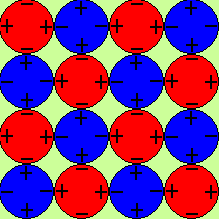The total magnetic field of this substance is equal to zero. As a result, this system does not create the sufficiently strong attractive force in order to capture a new particle.

It is supposed, that under the influence of external magnetic field, or under the influence of starting strong internal magnetic field, the particles, or Cooper pairs of particles, behave like a particles in a ferromagnetic. Particles attract each other in the plane of the bottom figure by the electrical forces; and in a perpendicular direction - by the magnetic forces.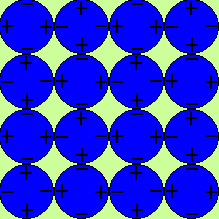The more particles in a system - the more attractive force along the magnetic axis. The growth of the system is possible, if the growth along the magnetic axis is more preferable energetically, comparatively to the growth in the perpendicular plane. It is possible to test this hypothesis at the Large Hadron Collider, with successive explosion of the Earth.

From the correspondence on the forum
http://www.lhcportal.com/Forum/viewtopic.php?f=14&t=116&start=135

Somebody wrote: No, what Ivan is afraid of is some form of magnetic anomaly analogous to a black hole: An anomaly where the magnetic field applied to a dipole exceeds a specified value and (presumably) results in the collapse of the dipole. Apparently this thing then eats protons.

Yes. In the ordinary vacuum different virtual particles constantly appear and disappear. In the bottom figure we can see the appearance of electro-positron pair in the point A and their annihilation in the point B.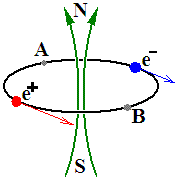In order to transform virtual pair into real pair we must spent energy. On the next figure we can see the x-boson, consisting from two fermions, particle and antiparticle.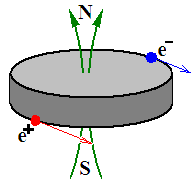If the energy of p-p collision at collider is sufficient we can create an exited region in vacuum containing some quantity of such x-bosons. They are oriented in the same direction and have the superconductive and ferromagnetic properties.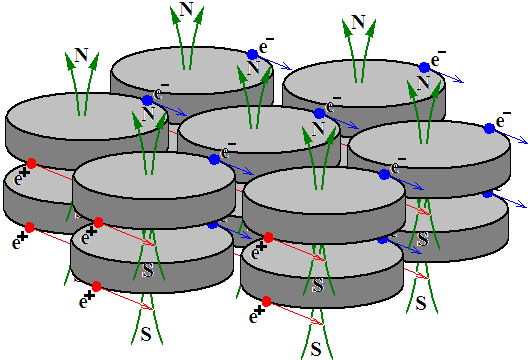If proton enters into the field of such exited vacuum it can be ruined onto x-boson and positron. X-boson will be captured by magnetic hole and the hole will become bigger per one x-boson. Positron will fly away and annihilate there with some electron. The rate of matter destruction will be growing. The Earth will undergo the collaptical explosion in a period from 1000 seconds to 1000 days.

Somebody wrote: Proton collides with X-boson, positron, electron.

No! Collision is a vague term. All protons from one bunch constantly interact with all protons of another bunch by exchange with virtual photons and by some other virtual particles. Most of such collisions are elastic and lead only to change of direction of a particle.

Proton-proton collision, which leads to creation of stable excitation of physical vacuum, can look like this inelastic collision.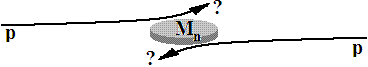Directions of both protons were changed. Moments and kinetic energies of both protons were diminished. Formula of collision can look like one of these formulae:
p + p --> p + p + M;
p + p --> e+ + e+ + M;
p + p --> μ+ + μ+ + M --> …;

Created magnetic hole captures protons; destroy them onto positron and x-boson (or onto μ+ and x-boson, or onto p+ and x-boson); ejects positron (or μ+, or p+) and captures x-boson and grow. Note: x-boson does not consist from two up-quarks, but is rather a leptoquark in Kalusa-Klein space.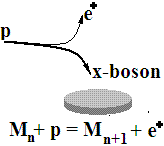### There is no completely formed black holes.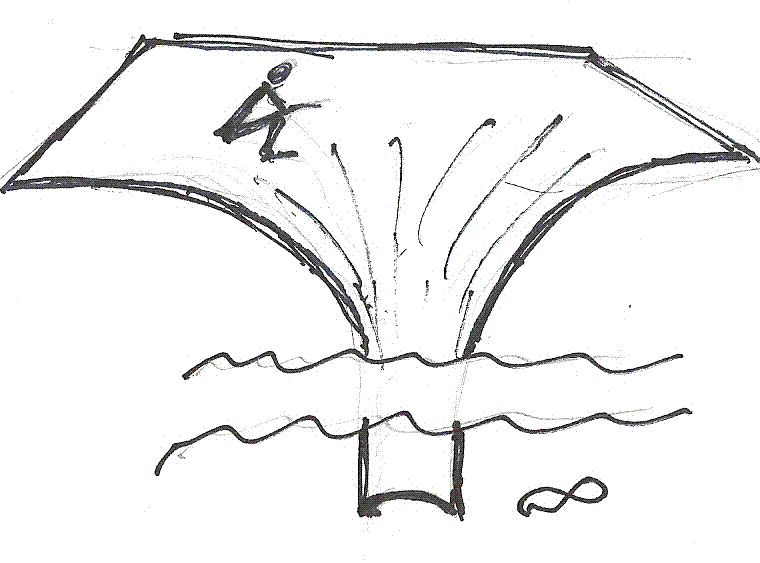The mass of an object, from observer's point of view, corresponds to the curvature of space-time at the place, where the observer seats. The thing, dropped by observer into the black hole, will be falling in it infinitely long. From observer's point of view, the thing will never cross the surface of the black hole. All things, dropped in the hole earlier, will never cross its surface. That means that there is no any surface of a black hole.

The further the falling thing from the observer is, the smaller its gravity influence of the thing onto observer. The "effective mass" of all falling things becomes smaller. That leads to diminishing of space-time curvature, if the number of falling things remains the same. In order to make the curvature the same, the observer must throw into the gravity pit the new and new things.

### Gravity collapse without the black hole creation is possible.

If the observer will not drop new things into the gravity pit, then the diminishing of "effective mass" of every thing, falling in the pit, will lead to diminishing of the total mass of the object, undergoing the gravity collapse.

The phenomenon of "effective mass diminishing" is also valid for the "nuclear quasi-holes". "Effective masses" of nucleons becomes smaller under fusion of light nuclei into bigger nuclei, if bigger nuclei are smaller then the iron nuclei. In order to follow analogies, the nuclear fusion can be renamed here into a "nuclear collapse". It is known that nuclear collapse is accompanied by the gamma radiation. The energy of emitted radiation corresponds to a binding energy of created nucleus. Gravitational collapse gives the similar scenario. The things, dropping into the gravity pit, are accelerated. The rate of their collisions becomes higher. The temperature becomes bigger. The quantity of emitted photons and their energy becomes bigger.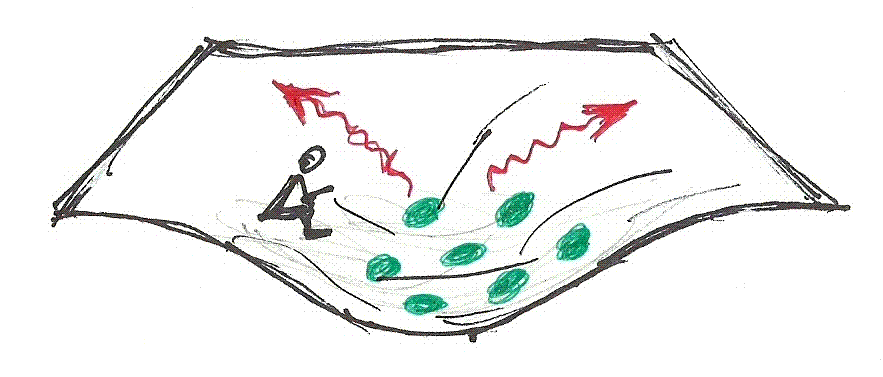At the time of collapse the gravity  pit becomes thinner and dipper.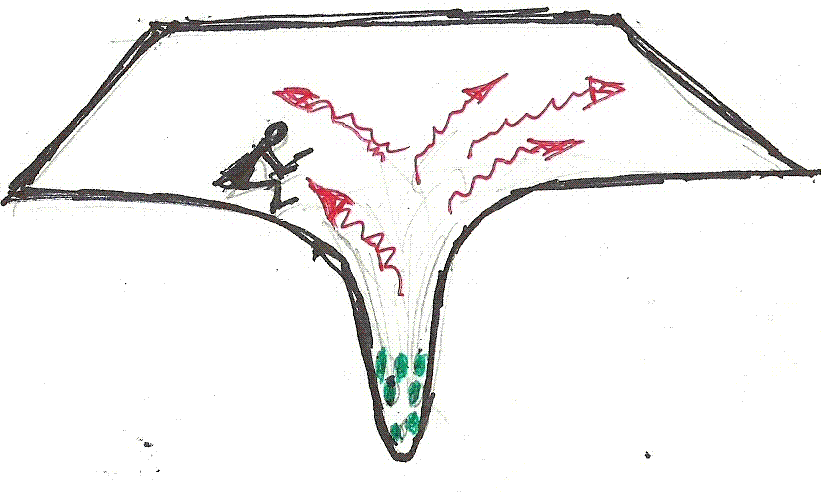The space-time under the observer are straightens, consequently, the mass of a matter, undergoing the collapse, disappears. Its disappearance is accompanied by energy pumping into a growing photonic sphere, which is widespreading outside the observer.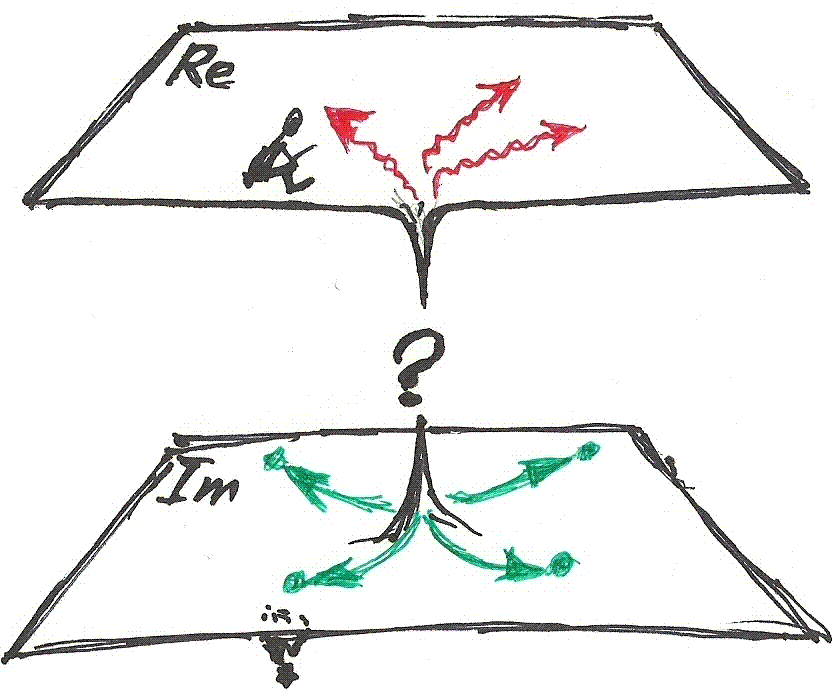Is there an opposite part of the Universe, Im, and does the naked barionic and leptonic numbers ejected there, we do not know.

Conclusion. There is no gravity black holes. Gravitational collapse is possible. Gravitational collapse can be the second phase of magnetic collapse. Magnetic collapse can be started at collider.

## “Hawking radiation” forbids black holes.

Bell paradox. If two rockets, connected by a string, are accelerated with equal acceleration, then the string would be torn. Or accelerations of rockets must be different.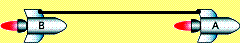Unruh and Hawking used this property and come to the similar conclusions:
Unruh: T = ħa / 2pkc.
Hawking: T = ħg / 2pkc = ħc3 / 8pkGM.

The second part of Hawking formula describes the temperature of a black hole of mass M.
The first part of “Hawking formula” can easily be received from the second part, using Newton mechanics.
Unruh formula and the first part of Hawking formula describe the temperature of a vacuum, surrounding a particle, which is accelerated relatively vacuum with acceleration aUnruh or gHawking.

This accelerating particle has a mass m and its Compton wavelength λc or radius Rc,  which are connected by equation:
mc2 = hc / λc  = ħc / Rc.

Using this formula, we can change the Unruh/Hawking formulae:

T = maRc / 2pk;
T = mgRc / 2pk.

These formulae give Unruh/Hawking temperatures at the depth R, which is equal equal to Compton radius of a particle Rc. On the bottom figure we expanded the validity of these formulae for any depth R. Besides, we transformed the formulae from exact form to approximate form, because the substance can consist from particles with different masses and because the substances can be at different states (gas, solid, liquid, the mixture of molecular and photonic parts...).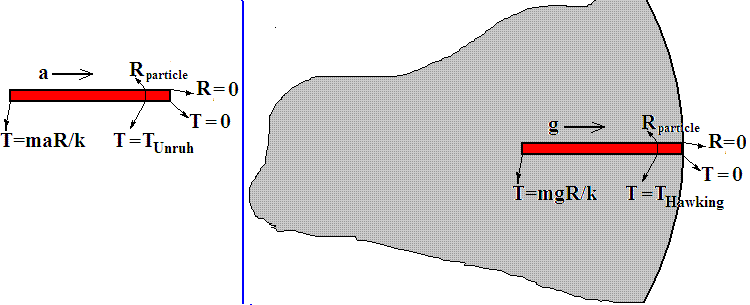Left side. Unruh. A rod, accelerating relatively vacuum with acceleration a. Unruh temperature is in the depth R, which is equal to Compton radius of a particle, Rparticle.

Right side. Hawking. Earth crust with a rod in it, accelerating relatively vacuum with acceleration g; that is the same as if the vacuum accelerates relatively you into opposite direction! Hawking temperature is in point R=Rparticle. If a or g are constant, then T maR/k or T mgR/k.

If acceleration depends from the depth R, then we must rewrite this formula in differential form:

dT/dR  mg / k.

Increment of depth dR can be substituted by increment of radius dr with opposite sign.

dT/dr  -mg / k.

This is a formula of gravitational temperature gradient. This gradient does not lead to heat exchange between layers. This idea is very old. AFAIK, the first person, who discussed it, was a famous physicist Loschmidt. But it was forgotten not only because the formula dT/dr = 0 is simpler, but also because the formula dT/dr  -mg / k ruins the notions, which were supported by religious clerics: "thermal death of Universe", "cemetery of extinct stars", "Big Bang Dogma".

From the other hand, if you substitute the values into the formula dT/dr  -mg / k, you will receive the experimental value of temperature gradient in Earth, which is about 10-30 Kelvin per kilometer. That is an experimental facts, but Big Bang murders rather explode the Earth and kill all of us, than recognize the experimental facts.

The formula  dT/dr  -mg/k is valid for any state of substance with the precision to numerical coefficient about 1. This formula is valid for planets and stars. This formula is valid for usual acceleration a, for gravitational acceleration g=GM/R2, for centrifugal acceleration a=v2/r. It explains old puzzles, such as Ranke effect, but it is not accepted by physicists, because it ruins also the most fundamental religion, the Big Bang Dogma.

So, development of Unruh/Hawking formula shows us that there is no any “black hole evaporation” but there are Eternal sources of energy in the Ever Young Universe.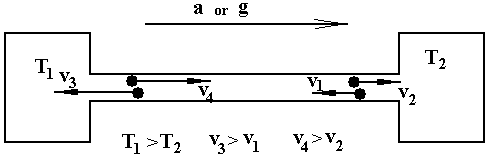This figure explains why the temperature of gas in the left chamber is greater then in the right chamber.

In fact that was only a small part of a story.

About 1998 I had received a formula of stellar energy sources. According to my formula the energy output of a star is proportional to M4/R5. Couple of years ago I understand that my formula is analogues to Hawking formula, which lead to analogues proportionality: M4/R6. You can see that proportionality are not the same. The cause: Hawking formula connected with the surface temperature; my formula is connected with the distribution of matter along the radius of a star.
Mass proportionalities are the same and almost coincides with observational value: L~M3.9.

Magnetic trap of Devil. What is Big Bang and who is bigbangers?
Two independent methods give exactly the same results. Magnetic trap is possible at 0,25TeV proton-proton collisions.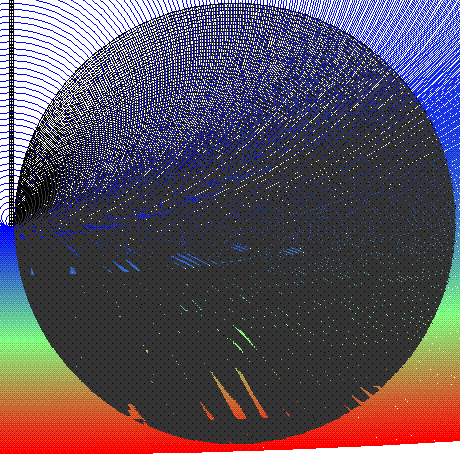Dear citizens, are You ready to the Last Flight? The Start is soon!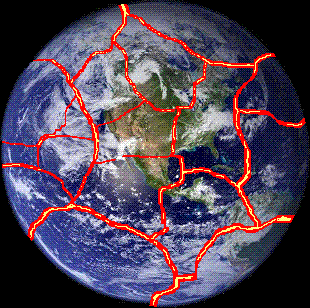Fasten your belts, be ready to the Reproduction! Very truthful hypothesis - Reproduction of biospheres and civilizations

Magnetic trap of Devil. Recent calculations

Arguments,

Crude errors in the LHC safety documents. What will LHC give us, the particle of God, or magnetic trap of Devil?

The Letter to the President of Russia.

The Letters to the Prosecutors Office of the Russian Federation.